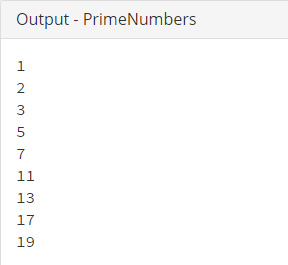##### Java Programming Examples Tutorial Index

This Java program demonstrates how to calculate and print prime numbers.

Example:

2, 3, 13 are prime numbers.

## Prime Number Check Program in Java

Example:

``````public class PrimeNumbers {

public static void main(String[] args) {

int num = 20, count;

for (int i = 1; i <= num; i++) {
count = 0;
for (int j = 2; j <= i / 2; j++) {
if (i % j == 0) {
count++;
break;
}
}

if (count == 0) {
System.out.println(i);
}

}
}
}``````

Program Output:Explanation:

First you have to create a class name PrimeNumbers inside which the main() method is declared. Now the main() method contains two integer type variables name - num and count. Variable num is initialized with the value 20.

Now, to check for all the integer numbers which is less than or equal to 20, you have to iterate the calculations for each value using a for loop.

The for loop statements:

``````for (int i = 1; i <= num; i++) {
count = 0;
for (int j = 2; j <= i / 2; j++) {
if (i % j == 0) {
count++;
break;
}
}``````

These statements are used to check whether i and j gives remainder as 0 (zero) or not. If the remainder comes as zero, count gets incremented by one. The next statement checks whether count is equal to zero or not. If zero, then prints the value of i.